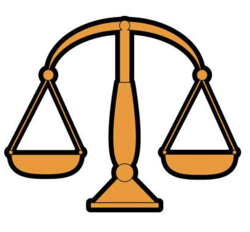#12 Balls Weight Puzzle (Solved)

This is my favorite weight puzzle which have been asked from me in many interviews over the past few years.

## Puzzle

You have 12 balls identical in size and appearance but 1 is an odd weight (could be either light or heavy).

You have a weighing scale with no measurements so you can just compare weight of balls against each other. You have only 3 chances to weigh the balls in any combination using the scales. Determine which ball is the odd one and if it’s heavier or lighter than the rest. How do you do it?

## Solution

First of all we will give a number to each ball i.e. 1, 2, 3, 4, 5, 6, 7, 8, 9, 10, 11, and 12

The trick to solve these kind of weight problem is to divide them in groups. We will divide these 12 balls in 3 groups:

GroupBall Numbers
group 11, 2, 3, 4
group 25, 6, 7, 8
group 39, 10, 11, 12

Now we keep the group 3 aside and put group 1 balls on one side of scale and group 2 balls on another side of scale  with three possible outcomes:-

#### 1. Scale is balanced

That means each ball in group 1 and group 2 are identical in weight and defective one is from group 3.

We know that defective one if from group 3 but we don’t know that defective one is lighter or heavier as compare to others. To solve this we need to weigh some balls from group 3 against some balls from either group 1 or group 2

Let’s weight group 3 (9, 10, 11) vs group 2 (6, 7, 8) with three possible outcomes:-

1. If group 3 (9, 10, 11) and group 2 (6, 7, 8) balances, then 12 is the odd ball. Weigh it against any other ball to determine if heavy or light.
2. If group 3 (9, 10, 11) is heavy then they contain a heavy ball. Weigh 9 vs 10, if balanced then 11 is the odd heavy ball, else the heavier of 9 or 10 is the odd heavy ball.
3. If group 3 (9, 10, 11) is light then they contain a light ball. Weigh 9 vs 10, if balanced then 11 is the odd light ball, else the lighter of 9 or 10 is the odd light ball.

#### 2. group 1 is heavier then group 2

That means either group 1 (heavy ball) or group 2 (light ball) contains the defective ball and group 3 has all good balls.

Let’s weigh 5,6,1 vs 7,2,12 with 3 possible outcomes:-

1. If 5,6,1 vs 7,2,12 balances, then either 8 is the odd light ball or 3 or 4 is the odd heavy ball. Weigh 3 vs 4, if they balance then 8 is the odd light ball, or the heaviest of 3 vs 4 is the odd heavy ball.
2. If 7,2,12 is heavy then either 2 is the odd heavy ball or 5 or 6 is the odd light ball. Weigh 5 vs 6, if balanced then 2 is the odd heavy ball, or the lightest of 5 vs 6 is the odd light ball.
3. If 7,2,12 is light then either 7 is light or 1 is heavy. Weigh 7 against any other ball, if balanced then 1 is the odd heavy ball else 7 is the odd light ball.

#### 3. group 2 is heavier then group 1

That means either group 2 (heavy ball) or group 1 (light ball) contains the defective ball and group 3 has all good balls.

Let’s weigh 1,2,5 vs 3,6,12 with 3 possible outcomes:-

1. If 1,2,5 vs 3,6,12 balances, then either 4 is the odd light ball or 7 or 8 is the odd heavy ball. Weigh 7 vs 8, if they balance then 4 is the odd light ball, or the heaviest of 7 vs 8 is the odd heavy ball.
2. If 3,6,12 is heavy then either 6 is the odd heavy ball or 1 or 2 is the odd light ball. Weigh 1 vs 2, if balanced then 6 is the odd heavy ball, or the lightest of 1 vs 2 is the odd light ball.
3. If 3,6,12 is light then either 3 is light or 5 is heavy. Weigh 3 against any other ball, if balanced then 5 is the odd heavy ball else 3 is the odd light ball.